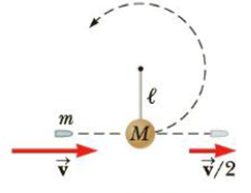Chapter 6, Problem 64AP

Chapter
Section
Textbook Problem

A bullet of mass m and speed v passes completely through a pendulum bob of mass M as shown in Figure P6.64. The bullet emerges with a speed of v/2. The pendulum bob is suspended by a stiff rod of length l and negligible mass. What is the minimum value of v such that the bob will barely swing through a complete vertical circle?Figure P6.64

To determine
The minimum value of v.

Explanation

Given info: Mass of the bullet is m. Speed of the bullet is v. Mass of the pendulum bob is M.

From law of conservation of energy, the kinetic energy at the bottom is equal to the potential energy at the top. Therefore,

12Mu2=Mg(2l)

• u is the velocity of the bob.

On Re-arranging,

u=2gl

On applying law of conservation of momentum,

mv=m(v2)+Mu

On Re-arranging,

Still sussing out bartleby?

Check out a sample textbook solution.

See a sample solution

The Solution to Your Study Problems

Bartleby provides explanations to thousands of textbook problems written by our experts, many with advanced degrees!

Get Started